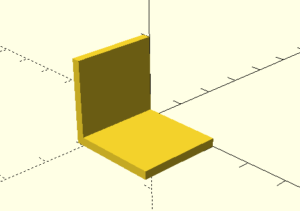# 3D Printing

Laurent software Tutorials and 3D models
Tutorials

## 2. Moving and changing our objects

### a. Translations

Now you know how to create shapes you maybe want to change their position in the view. It’s why we’ll use translate and rotate.

To move a shape you just need to do a translate([x,y,z]), example :

On the x axe :

`translate([10,0,0]) cube(10);`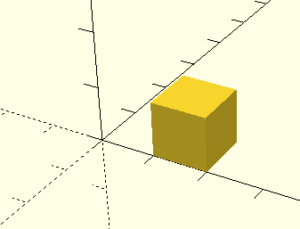On the y axe :

`translate([0,10,0]) cube(10);`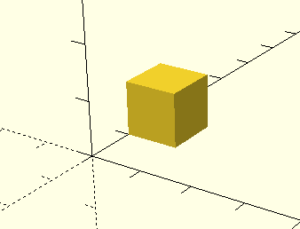On the z axe:

`translate([0,0,10]) cube(10);`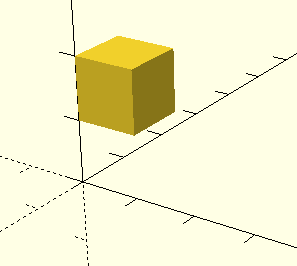```\$fn = 100;

translate([20,0,0]) cube(10);

translate([10,-10,0]) cylinder(10,5,5);;

translate([0,0,10]) sphere(r=5);```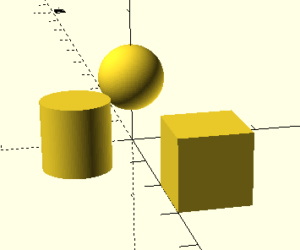### b. Rotations

The rotation is the same thing then translation instead we work with degrees (syntax : rotate([x,y,z])):

```\$fn = 100;

translate([20,0,0]) rotate([-30,0,0]) cube(10);

translate([10,-10,0]) rotate([90,0,0]) cylinder(10,5,5);;

translate([0,0,10]) sphere(r=5);```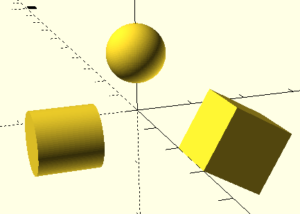The rotation is around an axe. Look at the cube we made a rotation of 30 degrees around the x axis but we go in the over direction with a -30 instead of just 30.

### c. Scale

A very interesting function when manipulating your shapes is scale(). Scaling will allow you to change the dimension of your shape by multiplication factors, example:

```translate([20,0,0]) cube(10);

scale([1,1.5,2]) cube(10);

scale([1,1.5,2]) //this function multiple x by 1, y by 1.5 and z by 2.```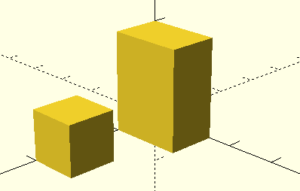### d. Exercise 2

Try to reproduce this shape (correction)